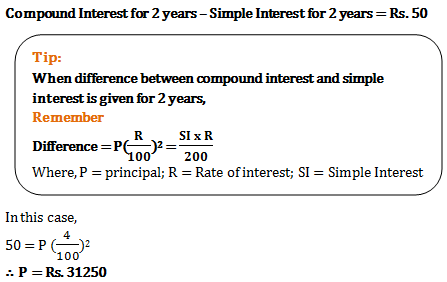# Compound Interest - Quantitative Aptitude (MCQ) questions for Q. 29497

Q.  Shamik had invested same amount of sums at simple as well as compound interest. The time period of both the sums was 2 years and rate of interest too was same 4% per annum. At the end, he found a difference of Rs. 50 in both the interests received. What were the sums invested?
- Published on 03 May 17

a. Rs. 32550
b. Rs. 35750
c. Rs. 30000
d. Rs. 31250